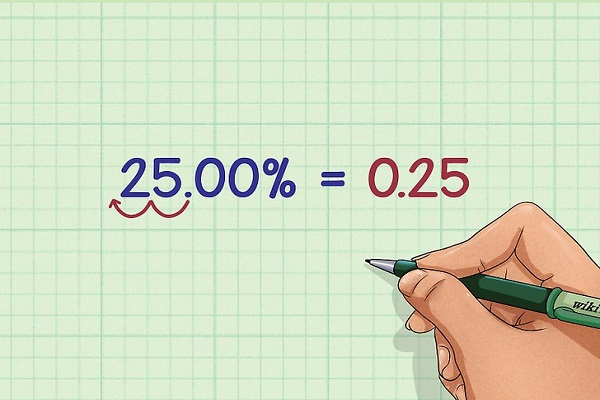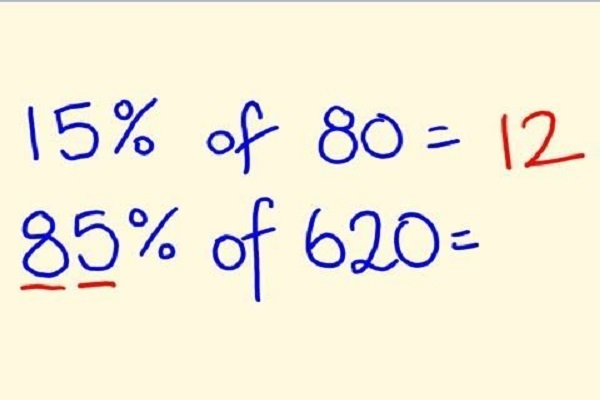# How to calculate percentages with and without calculators

You want to calculate percentages with or without the calculator but you do not know how to do (or you forgot). It is very simple, are almost all calculators now have special keys for this specific calculation. Also, take your sheet and pen, and take your calculator, while how you to calculate percentages with and without calculator teaches.

You may read also be interested: How to persevere in your studies

We used to illustrate this article the Windows calculator, but you can, of course, use any calculator.Here is an example to explain in a clear and simple way the best way to calculate a percentage with the calculator. Suppose we want to calculate 20% of 150. The first thing to do is write on the calculator the number on which we will apply the percentage, i.e. 150.

The next step is to multiply the previous quantity (by pressing the sign x or *) the figure of the percentage to be calculated, which in our example is 20.

For now, our operation is 150 x 20

Now, if your calculator has the key (%), it’s time to press it. Normally, all calculators, however simple they are. This feature allows you to calculate the percentage directly, without having to perform other calculations.

##Thus, following this example we will have on our calculator:

150 * 20%

By pressing the percentage key on your calculator, we will get the result directly on the screen, ie the percentage we wanted to calculate.

In our case, we can say that 20% of 150 are 30, as the calculator tells us.If you want to check that you have correctly calculated the percentage on your calculator, you can do the check by doing the calculation by hand. Calculate a percentage return to realize the rule of 3.

Thus, if we take our example, we can have the 100% that equals 150, and 20% equals x because we do not know its value. To find x, we have to multiply by crop and divide by the remaining number, in this case, 100 because it is a percentage.

By multiplying 20 x 150 and dividing by 100, we get the 20% of 150, which is 30, the same result obtained by the calculator.